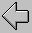Question…Can the Bible be true, even though it's not based on mathematics?

# September 18, 2001

A question came in from a vistor:

I had this one dude say that the logic is the language of mathematics and since the Bible is not based on mathematics, it cannot be true.

I replied:

1. Logic is NOT THE SAME as mathematics, nor is "mathematical logic" the only KIND of logic. There are several "types" of logic, and 'classical logic' extends WAY beyond mathematics, to supposedly ALL types of reasoning.

2. Math is a language of its own--logic is not necessarily its 'language' at all. when I write a series of simultaneous equations, and solve them using transformation rules, NONE of that is expressed in canonical logic forms (e.g. no syllogisms, no predicate calculus formulations).

3. Logic is about FORM and not about content--it is NOT about 'truth' at all, but rather 'validity' of form. Any logic textbook will explain this in the Introduction.

4. Logic is VERY LIMITED in what it can even 'talk about'--see my discussion in http://www.webcom.com/ctt/decidel.html, skip down to the section that begins with "I admit that I have no 'strictly logical' reason for seeking the truth. And I shudder at the thought that..."

5. MOST of what we know to be true has nothing to do with logic or mathematics, and therefore things can be 'true' without being mathematical at all. Consider these true, non-math, not-stated-in-logical-form statements:

a. "Mary speaks French."

b. "George Bush is the current president of the United States."

c. "Water molecules are composed of hydrogen and oxygen atoms."

d. "Logic deals with form and validity."

e. "Only math things are true."

f. "Jealously eats one's soul from the inside."

g. "Death happens to all"

h. "I am depressed"

i. "All colored objects are extended."

j. "All effects require causes"

k. "There are an infinite number of points within a circle of finite diameter"

This ought to be enough to show that the other fellow's statement is just too 'narrow' (as stated in your email) to represent the reality in which he/she/we live...The Christian ThinkTank...[http://www.Christian-thinktank.com] (Reference Abbreviations)